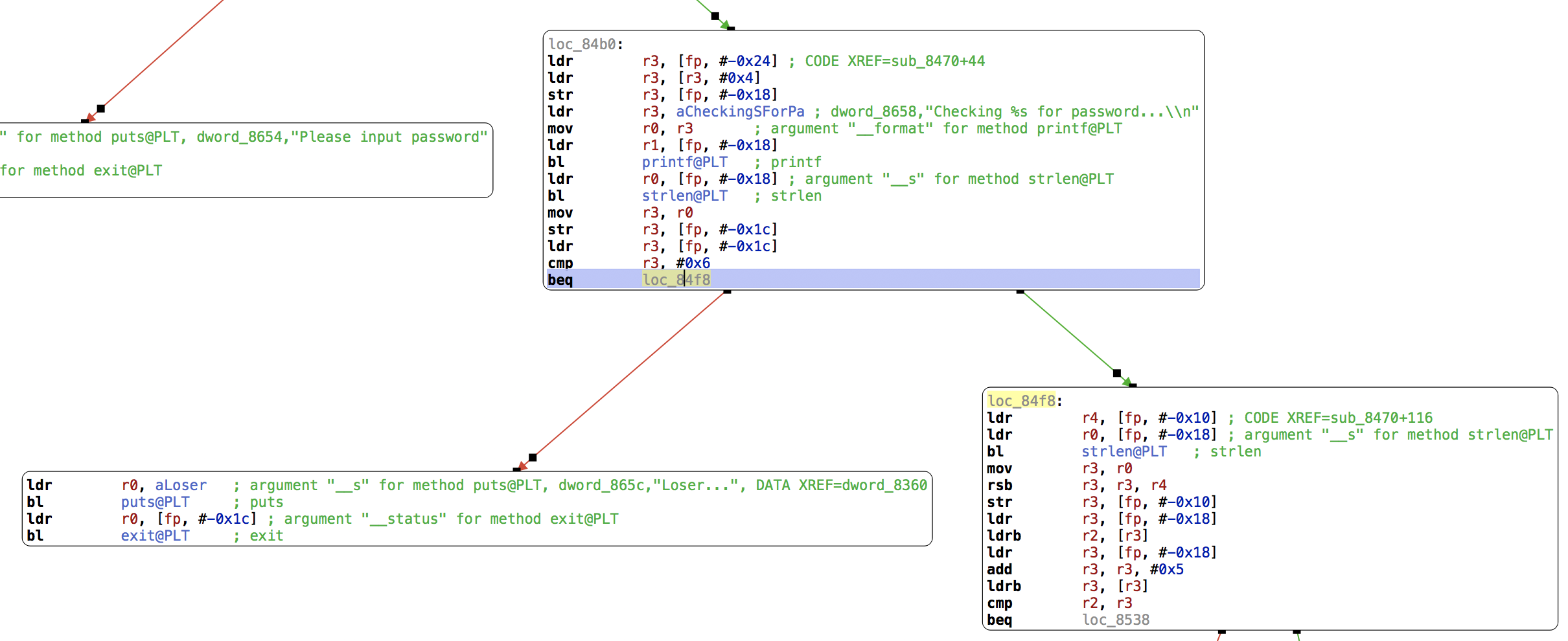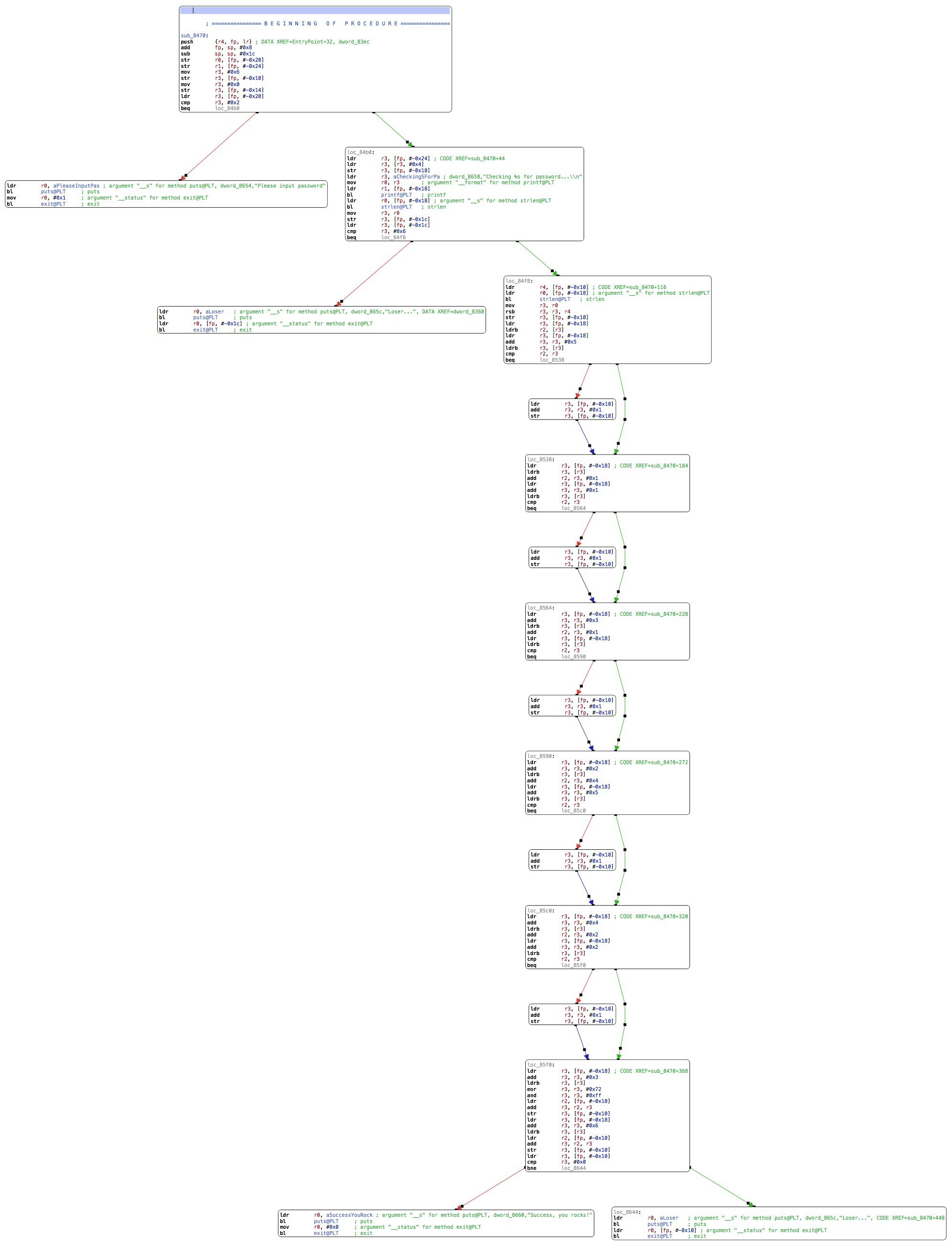# Crackme 5: ARM, basic

## Mar 14, 2018

First ARM binary! We won’t be able to run it in Docker either, so let’s dive in with Hopper.

Again, let’s follow the string “Checking %s for password…\n” and look at the logic around the code that references it:In this first block, `fp` is the frame pointer, the base address for local variables. The one at offset 0x18 is a string, passed to `printf` as the value for the “%s” entry mentionned above. For function calls with four or fewer 32-bit parameters, registers `r0` to `r3` are used to pass in the values. After a call, `r0` contains the return value. Here the format string is placed into `r0` via `r3` and the string at `fp` - 0x18 is referenced by `r1`. This is our input. `bl` is Branch-with-Link, similar to `call` in x86 assembly.

``````ldr        r3, aCheckingSForPa ; dword_8658,"Checking %s for password...\\n"
mov        r0, r3       ; argument "__format" for method printf@PLT
ldr        r1, [fp, #-0x18]
bl         printf@PLT   ; printf
``````

After the call to `printf`, we compute the input’s length, store it in a local variable at `fp` - 0x1c, and compare it to 6. We Branch-if-Equal (`beq`) to `loc_84f8`. If the length is not 6, we print an error message.

``````ldr        r0, [fp, #-0x18] ; argument "__s" for method strlen@PLT
bl         strlen@PLT   ; strlen
mov        r3, r0
str        r3, [fp, #-0x1c]
ldr        r3, [fp, #-0x1c]
cmp        r3, #0x6
beq        loc_84f8
``````

The password length is 6! Let’s look at the remaining validation code. The dump being pretty verbose, we’ll look at some of the assembly to get a general idea of the language before switching to Hopper’s pseudo-code view.

In the first block of this function, we had set the variable at `fp` - 0x10 (let’s call it `var_10`) to 6:

``````mov        r3, #0x6
str        r3, [fp, #-0x10]
``````

Now that we’ve checked the length, we use this local variable again and copy its value to `r4` before calling `strlen` again and saving that into `r3`:

``````loc_84f8:
ldr        r4, [fp, #-0x10] ; CODE XREF=sub_8470+116
ldr        r0, [fp, #-0x18] ; argument "__s" for method strlen@PLT
bl         strlen@PLT   ; strlen
mov        r3, r0
``````

`r3` now has the length, and `r4` is 6. We compute `r3 = r3 - r4` and put this back in `var_10`:

``````rsb        r3, r3, r4
str        r3, [fp, #-0x10]
``````

So we started with `var_10` = 6, subtracted the string length from it – which we know to be 6 – `var_10` is therefore zero at this point. We then load the first byte of our input into `r2`:

``````ldr        r3, [fp, #-0x18]
ldrb       r2, [r3]
``````

And the fifth byte of our input into `r3` (load address into `r3`, add 5, dereference into `r3`):

``````ldr        r3, [fp, #-0x18]
ldrb       r3, [r3]
``````

Compare `r2` and `r3`:

``````cmp        r2, r3
beq        loc_8538
``````

if they are equal, we jump over a block. This block contains the following code, which is simply incrementing `var_10`:

``````ldr        r3, [fp, var_10]
str        r3, [fp, var_10
``````

In effect, we ran:

``````var_10 = 0;
if (input != input) {
var_10++;
}
``````

There are several more similar comparisons following:They decode to:

``````if (input + 1 != input) {
var_10++;
}
if (input + 1 != input) {
var_10++;
}
if (input + 4 != input) {
var_10++;
}
if (input + 2 != input) {
var_10++;
}
``````

The next block is different. We start by loading `input` into `r3`:

``````loc_85f0:
ldr        r3, [fp, #-0x18] ; CODE XREF=sub_8470+368
ldrb       r3, [r3]
``````

We XOR `r3` with 0x72, AND with 0xff, and store the result back into `r3`:

``````eor        r3, r3, #0x72
and        r3, r3, #0xff
``````

We then load `var_10` into `r2`, add `r2` and `r3`, and put this whole thing back into `var_10`:

``````ldr        r2, [fp, #-0x10]
str        r3, [fp, #-0x10]
``````

We load `input` (which we know is a null byte) into `r3`, `var_10` into `r2`, add the two together and put the sum back into `var_10`:

``````ldr        r3, [fp, #-0x18]
ldrb       r3, [r3]
ldr        r2, [fp, #-0x10]
str        r3, [fp, #-0x10]
``````

We then compare `var_10` to zero and print the success message if they are equal:

``````ldr        r3, [fp, #-0x10]
cmp        r3, #0x0
bne        loc_8644
``````

Now that we have gone through this process manually, let’s examine the pseudo-code that Hopper generates for it. Click on the pseudo-code button, open the right frame and tick the “R11 based frame” check box. Navigate back to CFG mode and again to pseudo-code, which now displays:

``````int sub_8470(int arg0, int arg1) {
var_24 = arg1;
if (arg0 != 0x2) {
r0 = exit(0x1);
}
else {
var_18 = *(var_24 + 0x4);
var_1C = strlen(var_18);
if (var_1C != 0x6) {
puts("Loser...");
r0 = exit(var_1C);
}
else {
var_10 = 0x6 - strlen(var_18);
if (*(int8_t *)var_18 != *(int8_t *)(var_18 + 0x5)) {
var_10 = var_10 + 0x1;
}
if (*(int8_t *)var_18 + 0x1 != *(int8_t *)(var_18 + 0x1)) {
var_10 = var_10 + 0x1;
}
if (*(int8_t *)(var_18 + 0x3) + 0x1 != *(int8_t *)var_18) {
var_10 = var_10 + 0x1;
}
if (*(int8_t *)(var_18 + 0x2) + 0x4 != *(int8_t *)(var_18 + 0x5)) {
var_10 = var_10 + 0x1;
}
if (*(int8_t *)(var_18 + 0x4) + 0x2 != *(int8_t *)(var_18 + 0x2)) {
var_10 = var_10 + 0x1;
}
var_10 = var_10 + ((*(int8_t *)(var_18 + 0x3) ^ 0x72) & 0xff);
var_10 = var_10 + *(int8_t *)(var_18 + 0x6);
if (var_10 == 0x0) {
puts("Success, you rocks!");
r0 = exit(0x0);
}
else {
puts("Loser...");
r0 = exit(var_10);
}
}
}
return r0;
}
``````

This is readable and matches our analysis. We now have a full set of constraints that we can use to generate a valid input; since we know that `var_10` must always stay at zero, all the conditions need to match accordingly.

• The length must be 6.
• `input` == `input`
• `input` + 1 == `input`
• `input` + 1 == `input`
• `input` + 4 == `input`
• `input` + 2 == `input`
• (`input` ^ 0x72) & 0xff == 0
• `input` == 0

Starting from `input`, the only matching value that would be zero when XORed with 0x72 is 0x72 itself, which encodes the character ‘r’. From this we can deduce all the other values. The resulting password is the word “storms”.